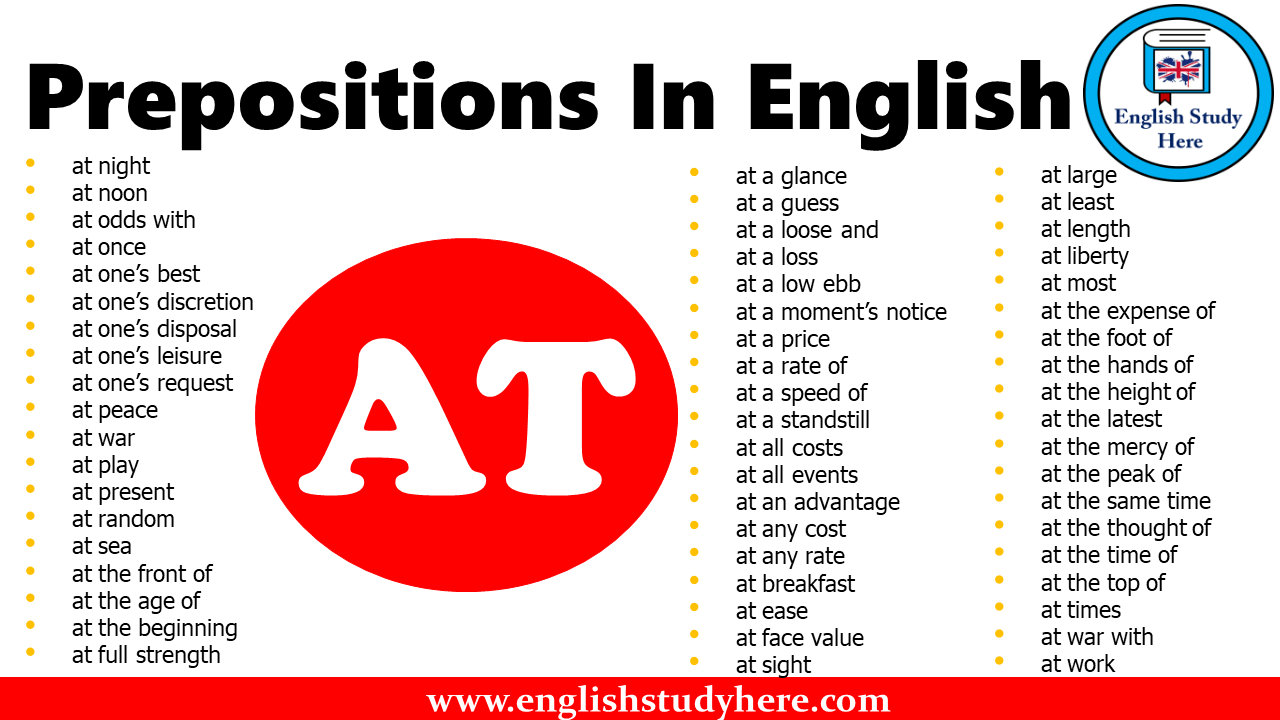# Prepositions In English – AT

Prepositions AT, Prepositions In English – AT, prepositions at• at night
• at noon
• at odds with
• at once
• at one’s best
• at one’s discretion
• at one’s disposal
• at one’s leisure
• at one’s request
• at peace
• at war
• at play
• at present
• at random
• at sea
• at the front of
• at the age of
• at the beginning
• at full strength

• at a glance
• at a guess
• at a loose and
• at a loss
• at a low ebb
• at a moment’s notice
• at a price
• at a rate of
• at a speed of
• at a standstill
• at all costs
• at all events
• at any cost
• at any rate
• at breakfast
• at ease
• at face value
• at sight
• at large
• at least
• at length
• at liberty
• at most
• at the expense of
• at the foot of
• at the hands of
• at the height of
• at the latest
• at the mercy of
• at the peak of
• at the same time
• at the thought of
• at the time of
• at the top of
• at times
• at war with
• at work